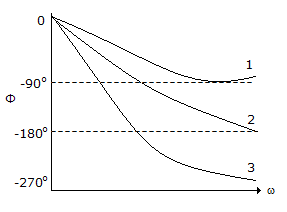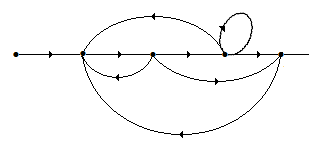# Electronics and Communication Engineering - Automatic Control Systems

### Exercise :: Automatic Control Systems - Section 9

1.

Which of the following is used to obtain output position in a position control system?

 A. Strain gauge B. Load cell C. Synchro D. Thermistor

Explanation:

No answer description available for this question. Let us discuss.

2.

In a synchro transmitter-control transformer, the voltage induced in rotor winding of control transformer is zero when the angular displacement between rotors of transmitter and transformer is

 A. 0° B. 90° C. 180° D. either (a) or (c)

Explanation:

No answer description available for this question. Let us discuss.

3.

In the given figure, the curves 1, 2, 3 are respectively forA. all pass, minimum phase and non-minimum phase functions B. minimum phase, all pass and non-minimum phase functions C. non-minimum phase, all pass and minimum phase functions D. minimum phase, non-minimum phase and all pass functions

Explanation:

No answer description available for this question. Let us discuss.

4.

In the given figure, the number of individual loops isA. 2 B. 4 C. 5 D. 6

Explanation:

No answer description available for this question. Let us discuss.

5.

DC tachogenerator can be used in

 A. speed control system B. position control system C. both (a) and (b) D. neither (a) nor (b)